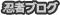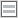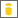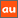# 0除算に関するアナウンスメントを公開しています

The purpose of this site is to publish announcements on the theory of division by zero

## Announcement 354: What are $n = 2,1,0$ regular polygons inscribed in a disc? -- relations of $0$ and $\infty$

Institute of Reproducing Kernels
Kawauchi-cho, 5-1648-16,
Kiryu 376-0041, Japan
February 7, 2017

Abstract: In this announcement,  as the representation of the point at infinity of the Riemann sphere by the zero $z = 0$, we will show its geometrical interpretation by $n$ regular polygons inscribed in a disc. This will show a delicate relation between $0$ and $\infty$. We will furthermore give surprising relations $0$ and $\infty$ which show a strong discontinuity at the point of infinity on the Riemann sphere.

1 Introduction

By a natural extension of the fractions
\begin{equation}
\frac{b}{a} \tag{1.1}
\end{equation}for any complex numbers $a$ and $b$, we found the simple and beautiful result, for any complex number $b$
\begin{equation}
\frac{b}{0}=0, \tag{1.2}
\end{equation}incidentally in  by the Tikhonov regularization for the Hadamard product inversions for matrices, and we discussed their properties and gave several physical interpretations on the general fractions in  for the case of real numbers. Meanwhile, the result (1.2) is a very special case of very general fractional functions in .
We thus should consider, for any complex number $b$, as  (1.2); that is, for the mapping
\begin{equation}
W = \frac{1}{z}, \tag{1.3}
\end{equation}
the image of $z=0$ is $W=0$ $($should be defined$)$. This fact seems to be a curious one in connection with our well-established popular image for the  point at infinity on the Riemann sphere. Therefore, the division by zero will give great impacts to complex analysis and to our ideas for the space and universe.
However, for functions, we will need some modification by the idea of the division by zero calculus:
For any formal Laurent expansion around $z=a$,
\begin{equation}
f(z) = \sum_{n=-\infty}^{\infty} C_n (z - a)^n, \tag{1.4}
\end{equation}
we obtain the identity, by the division by zero
\begin{equation}
f(a) =  C_0. \tag{1.5}
\end{equation}
The typical example is that, as we can derive by the elementary way,
$$\tan \frac{\pi}{2} =0.$$
We gave  many examples with geometric meanings in . See [1, 2, 3, 6, 7] for the related references.In ,  many division by zero results in Euclidean spaces are given and  the basic idea at the point at infinity should be changed. In , we gave beautiful geometrical interpretations of determinants from the viewpoint of the division by zero. The results show that the division by zero is our basic and elementary mathematics in our world.
For our ideas on the division by zero, see the survey style announcements stated in the references.

2  $n=0,1,2$ regular polygons inscribed in a disc

We consider $n$ regular polygons inscribed in a fixed disc with radius $a$. Then we note that their area $S_n$ and the lengths $L_n$ of the sum of the sides  are given by
\begin{equation}
S_n= \frac{n a^2}{2} \sin \frac{2\pi}{n}\tag{2.1}
\end{equation}
and
\begin{equation}
L_n= 2n a \sin \frac{\pi}{n},\tag{2.2}
\end{equation}
respectively. For $n \ge 3$, the results are clear.
For $n =2$, we will consider two diameters that are the same. We can consider it as a generalized regular polygon inscribed in the disc as a degenerate case. Then, $S_2 =0$ and $L_2 =4a$,  and the general formulas are  valid.
Next, we will consider the case $n=1$. Then the corresponding regular polygon is a just diameter of the disc. Then, $S_1 =0$ and $L_1 = 0$ that will mean that any regular polygon inscribed in the disc may not be formed and so its area and  length of the side are zero.
Now we will consider the case $n=0$. Then, by the division by zero calculus, we obtain that $S_0= \pi a^2$ and $L_0 = 2\pi a$. Note that they are the area and the length of the disc.  How to understand the results? Imagine contrary $n$ tending to infinity, then the corresponding regular polygons inscribed in the disc tend to the disc. Recall our new idea that the point at infinity is represented by $0$. Therefore, the results say that $n=0$ regular polygons are $n= \infty$ regular polygons inscribed in the disc in a sense and they are the disc. This is our interpretation of the theorem:

Theorem.   $n =0$ regular polygons inscribed in a disc are the whole disc.

3  Our life figure

As an interesting figure which shows an interesting relation between $0$ and infinity, we will consider a sector $\Delta_{\alpha}$ on the complex $z= x + iy$ plane
$$\Delta_{\alpha} = \left\{ |\arg z| < \alpha; 0< \alpha < \frac{\pi}{2}\right\}.$$
We will consider a disc inscribed in the sector $\Delta_{\alpha}$ whose center $(k,0)$ with radius $r$. Then, we have
\begin{equation}
r = k \sin \alpha. \tag{3.1}
\end{equation}
Then, note that as $k$ tends to zero, $r$ tends to zero, meanwhile $k$ tends to $+\infty$, $r$ tends to $+\infty$. However, by our division by zero calculus, we see that immediately that
\begin{equation}
[r ]_{r =\infty}= 0.\tag{3.2}
\end{equation}
For this fact, note the following:
The behavior of the space around the point at infinity may be considered by that of the origin by the linear transform $W = 1/z$. We thus see that
\begin{equation}
\lim_{z \to \infty} z = \infty,\tag{3.3}
\end{equation}
however,
\begin{equation}
[z]_{z =\infty} =0,\tag{3.4}
\end{equation}
by the division by zero. Here, $[z]_{z =\infty}$ denotes the value of the function $W=z$ at the topological point at the infinity in one point compactification by Aleksandrov. The difference of (3.3) and (3.4) is very important as we see clearly by the function $W = 1/z$ and the behavior at the origin. The limiting value to the origin and the value at the origin are different. For  surprising results, we will state the property in the real space as follows:
\begin{equation}
\lim_{x\to  +\infty} x =+\infty , \quad \lim_{x\to  -\infty} x = -\infty,\tag{3.5}
\end{equation}
however,
\begin{equation}
[x]_{ +\infty } =0, \quad  [x]_{ -\infty } =0.\tag{3.6}
\end{equation}
Of course,   two points  $+\infty$ and $-\infty$ are the same point as the point at infinity. However, $\pm$ will be convenient in order to show the approach directions. In \cite{mos}, we gave many examples for this property.
On the sector, we see that from the origin as the point 0, the inscribed discs are increasing endlessly, however their final disc reduces to the origin suddenly -  it seems that the whole process looks like our life in the viewpoint of our initial and final.

4  H. Okumura's example

The suprising example by H. Okumura which was sent to the Institute on 2016.12.1.9:40 will show a new phenomenon at the point at infinity.
On the sector  $\Delta_{\alpha}$, we shall change the angle and we consider a fixed circle $C_a, a > 0$ with radius $a$  inscribed in the sectors. We see that when the circle tends to $+\infty$, the angles $\alpha$ tend to zero. How will be the case $\alpha = 0$?  Then, we will not be  able to see the position of the circle. Surprisingly enough, then the circle $C_a$ is the circle with center at the origin $0$. This result is derived from the division by zero calculus for the formula
\begin{equation}
k =\frac{a}{\sin \alpha}.\tag{4.1}
\end{equation}
The two lines $\arg z = \alpha$ and $\arg z = -\alpha$ were tangential lines of the circle  $C_a$ and now they are the positive real line. The gradient of the positive real line is of course zero. Note here that the gradient of the positive imaginary line is zero by the division by zero calculus that means $\tan \frac{\pi}{2} =0$. Therefore, we can understand that the positive real line is still a tangential line of the circle $C_a$.
This will show some great relation between zero and infinity. We can see some mysterious property around the point at infinity.

References

 L. P.  Castro and S. Saitoh,  Fractional functions and their representations,  Complex Anal. Oper. Theory 7 $($2013$)$, no. 4, 1049-1063.
 M. Kuroda, H. Michiwaki, S. Saitoh, and M. Yamane,
New meanings of the division by zero and interpretations on $100/0=0$ and on $0/0=0$,
Int. J. Appl. Math.   27 $($2014$)$, no 2, pp. 191-198,  DOI: 10.12732/ijam.v27i2.9.
 H. Michiwaki, S. Saitoh,  and  M.Yamada,
Reality of the division by zero $z/0=0$.  IJAPM  International J. of Applied Physics and Math.  6$($2015$)$, 1--8. http://www.ijapm.org/show-63-504-1.html
 T. Matsuura and S. Saitoh, Matrices and division by zero $z/0=0$, Advances in Linear Algebra & Matrix Theory, 6 $($2016$)$, 51-58. http://dx.doi.org/10.4236/alamt.2016.62007 http://www.scirp.org/journal/alamt
 H.  Michiwaki, H. Okumura, and S. Saitoh, Division by Zero $z/0 = 0$ in Euclidean Spaces.  International Journal of Mathematics and Computation Vol. 28$($2017$)$; Issue  1,  1-16.
 S. Saitoh, Generalized inversions of Hadamard and tensor products for matrices,  Advances in Linear Algebra & Matrix Theory.  4  $($2014$)$, no. 2,  87--95. http://www.scirp.org/journal/ALAMT/
 S. Saitoh, A reproducing kernel theory with some general applications,
Qian,T./Rodino,L.$($eds.$)$: Mathematical Analysis, Probability and Applications - Plenary Lectures: Isaac 2015, Macau, China, Springer Proceedings in Mathematics and Statistics,   177$($2016$)$, 151-182 $($Springer$)$.
 Announcement 179 $($2014.8.30$)$: Division by zero is clear as z/0=0 and it is fundamental in mathematics.
 Announcement 185 $($2014.10.22$)$: The importance of the division by zero $z/0=0$.
 Announcement 237 $($2015.6.18$)$:  A reality of the division by zero $z/0=0$ by  geometrical optics.
 Announcement 246 $($2015.9.17$)$: An interpretation of the division by zero $1/0=0$ by the gradients of lines.
 Announcement 247 $($2015.9.22$)$: The gradient of y-axis is zero and $\tan (\pi/2) =0$ by the division by zero $1/0=0$.
 Announcement 250 $($2015.10.20$)$: What are numbers? -  the Yamada field containing the division by zero $z/0=0$.
 Announcement 252 $($2015.11.1$)$: Circles and curvature - an interpretation by Mr. Hiroshi Michiwaki of the division by zero $r/0 = 0$.
 Announcement 281 $($2016.2.1$)$: The importance of the division by zero $z/0=0$.
 Announcement 282 $($2016.2.2$)$: The Division by Zero $z/0=0$ on the Second Birthday.
 Announcement 293 $($2016.3.27$)$:  Parallel lines on the Euclidean plane from the viewpoint of division by zero 1/0=0.
 Announcement 300 $($2016.05.22$)$: New challenges on the division by zero z/0=0.
 Announcement 326 $($2016.10.17$)$: The division by zero z/0=0 - its impact to human beings through education and research.
 Announcement 352 $($2017.2.2$)$:   On the third birthday of the division by zero z/0=0.

PR

### コメント

お名前 Black LimeGreen SeaGreen Teal FireBrick Tomato IndianRed BurlyWood SlateGray DarkSlateBlue LightPink DeepPink DarkOrange Gold DimGray Silverプロフィール

ＨＮ：

(01/17)
(01/14)
(01/12)
(01/04)
(01/03)